# Hardest colleges to get into in Oregon

Top 10 colleges in Oregon with the lowest acceptance rates
What are the hardest colleges to get into in Oregon? We've got you covered. We've compiled a national college database and have created a list of the hardest universities to get into in Oregon below. These are the hardest 4 year colleges to get into in Oregon, and sometimes some of the hardest colleges to get into in the US. You could even say these are the best colleges in Oregon. We also include each college's average SAT and ACT scores so that you can see where you're most competitive. Read on to find out more.

## Reed College acceptance rate

Reed College acceptance rate is 35%.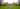The average SAT score for Reed College is 1420.

• SAT composite: 1420
• SAT math: 710

The average ACT score for Reed College is 32.

## Concordia University Portland Oregon acceptance rate

Concordia University Portland Oregon acceptance rate is 53.8%.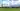The average SAT score for Concordia University Portland Oregon is 1070.

• SAT composite: 1070
• SAT math: 530

The average ACT score for Concordia University Portland Oregon is 22.

## University of Portland acceptance rate

University of Portland acceptance rate is 61.9%.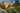The average SAT score for University of Portland is 1260.

• SAT composite: 1260
• SAT math: 620

The average ACT score for University of Portland is 27.

## Warner Pacific College acceptance rate

Warner Pacific College acceptance rate is 62.8%.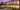The average SAT score for Warner Pacific College is 990.

• SAT composite: 990
• SAT math: 480

The average ACT score for Warner Pacific College is 18.

## Lewis and Clark College acceptance rate

Lewis and Clark College acceptance rate is 73.8%.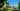The average SAT score for Lewis and Clark College is 1303.

• SAT composite: 1303
• SAT math: 640

The average ACT score for Lewis and Clark College is 28.

## Southern Oregon University acceptance rate

Southern Oregon University acceptance rate is 78.2%.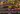The average SAT score for Southern Oregon University is 1100.

• SAT composite: 1100
• SAT math: 530

The average ACT score for Southern Oregon University is 22.

## George Fox University acceptance rate

George Fox University acceptance rate is 78.3%.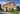The average SAT score for George Fox University is 1150.

• SAT composite: 1150
• SAT math: 560

The average ACT score for George Fox University is 23.

## Pacific University Oregon acceptance rate

Pacific University Oregon acceptance rate is 79%.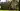The average SAT score for Pacific University Oregon is 1150.

• SAT composite: 1150
• SAT math: 570

The average ACT score for Pacific University Oregon is 24.

## Oregon State University acceptance rate

Oregon State University acceptance rate is 80.6%.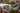The average SAT score for Oregon State University is 1195.

• SAT composite: 1195
• SAT math: 600

The average ACT score for Oregon State University is 25.

## Western Oregon University acceptance rate

Western Oregon University acceptance rate is 80.6%.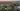The average SAT score for Western Oregon University is 1040.

• SAT composite: 1040
• SAT math: 510